﻿ ZetaPolynomials

## Zeta Polynomials and Harmonic Numbers

#### Peter Luschny, April 2010

KEYWORDS: Bernoulli Polynomials, Stirling Polynomials, Zeta Polynomials
Concerned with sequences: A109822, A064538, A135517

### ::: Harmonic numbers :::

The harmonic numbers are defined as

H := proc(n) local i;
if n = 0 then 1 else add(1/i, i=1..n) fi end:


There are several ways to generalize these numbers to continuous objects. A popular one is to define a function h(x) such that h(n) = H(n). For instance Sumk≥1(1/k−1/(k+z)) converges for all complex values of z, except when z is a negative number.

Another possibility is to define a sequence of polynomials hn(x) and evaluate this sequence at a fixed point a, hn(a) = H(n). We will look here at the polynomial approach. Consider the following sequence of polynomials

h := proc(n, x) local k, T;

T := proc(n, k) option remember;
if n = 0 then 1 elif k < 0 then 0 else
T(n−1,k)+(n−1)*T(n−1,k−1) fi end:

if n = 0 then 1 else add(T(n,k)*x^(n−k−1),k=0..n−1) fi end:
 n hn(x) 0 1 1 1 2 x+2 3 x2+4x+6 4 x3+7x2+18x+24 5 x4+11x3+46x2+96x+120

The coefficients are in A109822. An explicit formula is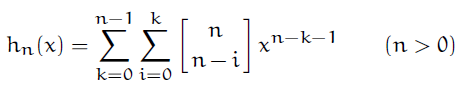Here [n over k] denote the signless Stirling numbers of the first kind.

plot([seq( h(n, x) / n!, n=0..6)], x=0..2);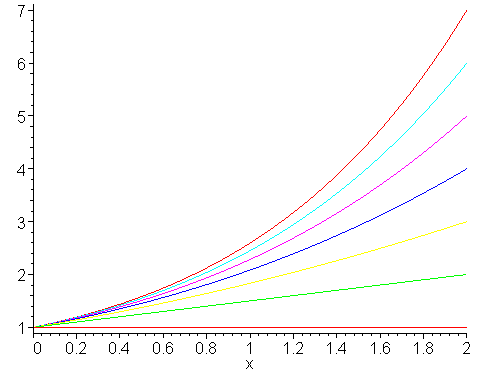The point of interest are the values of these polynomials at x=1.

 n hn(1) n! H(n) 0 1 0! 1 1 1 1! 1 2 1+2 2! (3/2) 3 1+4+6 3! (11/6) 4 1+7+18+24 4! (25/12) 5 1+11+46+96+120 5! (137/60) 6 1+16+101+326+600+720 6! (49/20)

It is a pity that these polynomials are not named harmonic polynomials. However this name is traditionally reserved for some other kind of mathematical objects.

### ::: Zeta polynomials :::

In my last blog I defined a sequence to sequence transformation

Ts := proc(s, n, x) local k, v;
add(add((-1)^v*binomial(k,v)*s(k+1)*(x+v+1)^n,v=0..k),k=0..n) end:

However, nothing prevents me to feed a sequence of functions into this transformation. The minor change this needs is to add 'x' to the parameter list of s.

Tf := proc(f, n, x) local k, v;
add(add((-1)^v*binomial(k,v)*f(k+1,x)*(x+v+1)^n,v=0..k),k=0..n) end:

Now we can easily define the transform of a sequence of polynomials. For instance, assuming the function hn(x) as the 'harmonic' polynomials defined above we set the zeta polynomials ζn(x) = Tf(hn(x)/n!).

ZetaPolynomial := proc(n, x) global h; Tf((n,x) -> h(n, x)/n!, n, x) end;

A first impression give the following plots. The zeta polynomials have a sharp focus at (0,0) and (2,1).And at midway, at x = 1? Let us first introduce some convenient abbreviations.

with(numtheory);
# Now we can write B(n,x) instead of bernoulli(n,x).

BP := proc(m, x) B(n, x/2+1/2) end;
alias(ZP = ZetaPolynomial):
# Now we can write ZP(n,x) instead of ZetaPolynomial(n,x).

seq(ZP(n,1), n=0..12);
1, 1/2, 1/6, 0, -1/30, 0, 1/42, 0, -1/30, 0, 5/66, 0, -691/2730,...

OMG! These are the Bernoulli numbers!

seq(eval(diff(ZP(n,x),x),x=1),n=3..13);
seq(eval(diff(ZP(n,x),x),x=0)*(-(n+1)/2),n=2..12);
1/4, 0, -1/12, 0, 1/12, 0, -3/20, 0, 5/12, 0, -691/420,...


Hmm, same as seq(eval(diff(BP(n,x),x), x=1), n=3..13). What is going on here? Am I reinventing the Bernoulli polynomials? One more test.

dcp1 := n -> denom(ZP(n, x+1) − ZP(n,1)):
dcp2 := n -> denom(ZP(n, x+1)):

Both sequences give

1, 2, 6, 4, 30, 12, 42, 24, 90, 20, 66, 24, 2730, 420, 90, 48, 510, 180, 3990,...

This is our old friend A064538, the smallest number a(n) such that a(n)*(1n + 2n +...+ xn) is a polynomial in x with integer coefficients. A064538 := n −> denom((Bn+1(x+1) − Bn+1(1))/(n+1)). However, this time A064538(n) simply equals denom(ζn(x)). This behavior of the zeta polynomials is agreeable different from the behavior of the Bernoulli polynomials, whose denom(Bn(x+1) − Bn(1)) differs from denom(Bn(x+1)).

Other interesting sequences in this context are related to the Clausen numbers.

Clausen := proc(n) local S, m;
S := divisors( n );
S := map(i -> i + 1, S);
S := select(isprime, S);
mul(m, m=S) end:


Clausen numbers divide the denominators of the zeta polynomials.

seq(denom(ZP(i,x))/Clausen(i), i=0..20);


1, 1, 1, 2, 1, 6, 1, 12, 3, 10, 1, 12, 1, 210, 15, 24, 1, 90, 5, 420, 21,..

For n = 3 + 2k (k≥0) the denominator of the zeta polynomials is divided by the square of the Clausen numbers.

1, 3, 6, 5, 6, 105, 12, 45, 210, 165, 180, 273, 14, 15, 1848, 1785,..


This pattern continues and we are let to the sequence

seq(gcd(denom(ZP(k,x)),2^k)/2, k=1..100);
1, 1, 2, 1, 2, 1, 4, 1, 2, 1, 4, 1, 2, 1, 8, 1, 2, 1, 4, ...


Look this sequence up at OEIS, we need it soon again. Next let us examine the zeta polynomials at x = 1/2.

seq((2^i*ZP(i,1/2)), i=0..17);
1, 1/2, 0, -1/4, 0, 1/2, 0, -17/8, 0, 31/2, 0, -691/4, 0, 5461/2,.. 

First at the denominator of ζi(1/2). To this end let

isPow2 := i -> if factorset(i) = {2} then 1 else 0 fi;
denE   := i -> (1+isPow2(i+1))*denom(euler(i,x+1)-euler(i,1));
denZP  := i -> denom(2^i*ZP(i,1/2));


Then by S.B.E's verification technique seq(denE(n) − denZP(n), n = 0..100)  = 0,0,... we see that denE(n) = denZP(n).  Without the factor  (1 + isPow2(n+1)) denE is Guy Steele's sequence GS(2,5), which is A135517(n) = 2^(A091090(n)−1).

Now the nominator of of ζn(1/2). The search engine of OEIS quickly leads me to a comment from N.J.A. Sloane: "(-1)^n*A002425 is the numerator of Euler(2n+1, 1)".  This can also be written as:

seq(numer(2^i*ZP(i,1/2)), i=0..21);
seq(numer((-1)^i*euler(i,0)), i=0..21);
1, 1, 0, -1, 0, 1, 0, -17, 0, 31, 0, -691, 0, 5461, ...


It seems that the following sequence is missing in the OEIS

seq(numer(ZP(2*i+1,3/2)),  i=0..11);
seq(numer(euler(2*i+1,2)), i=0..11);
3, 9, 3, 33, -27, 699, -5457, 929601, -3202287, 221930589,...


However, it is better to divide the sequence by 3 and add the following sequence

seq(abs(numer(ZP(2*i+1, -1/2))), i=0..11);
1, 3, 1, 11, 9, 233, 1819, 309867, 1067429, 73976863,...


As a last example we note

seq((-1)^i*3*ZP(i,-2),i=0..12);
3, 3, 5, 9, 17, 33, 65, 129, 257, 513, 1025, 2049, 4097, ...
A000051 = 2,3,5,9,17,33,65,129,257,513,1025, 2049, 4097,...


### ::: Zeta polynomials versus Bernoulli polynomials :::

Next let us get some visual aid. Since Bn(1 − x) = (−1)n Bn(x) we can restrict our study to (1/2,1). For convenience we rescale this restriction in the plots to (0,1), thus we look at Bn(x/2+1/2). Zooming into the interval [0,1] and comparing with these Bernoulli polynomials we see: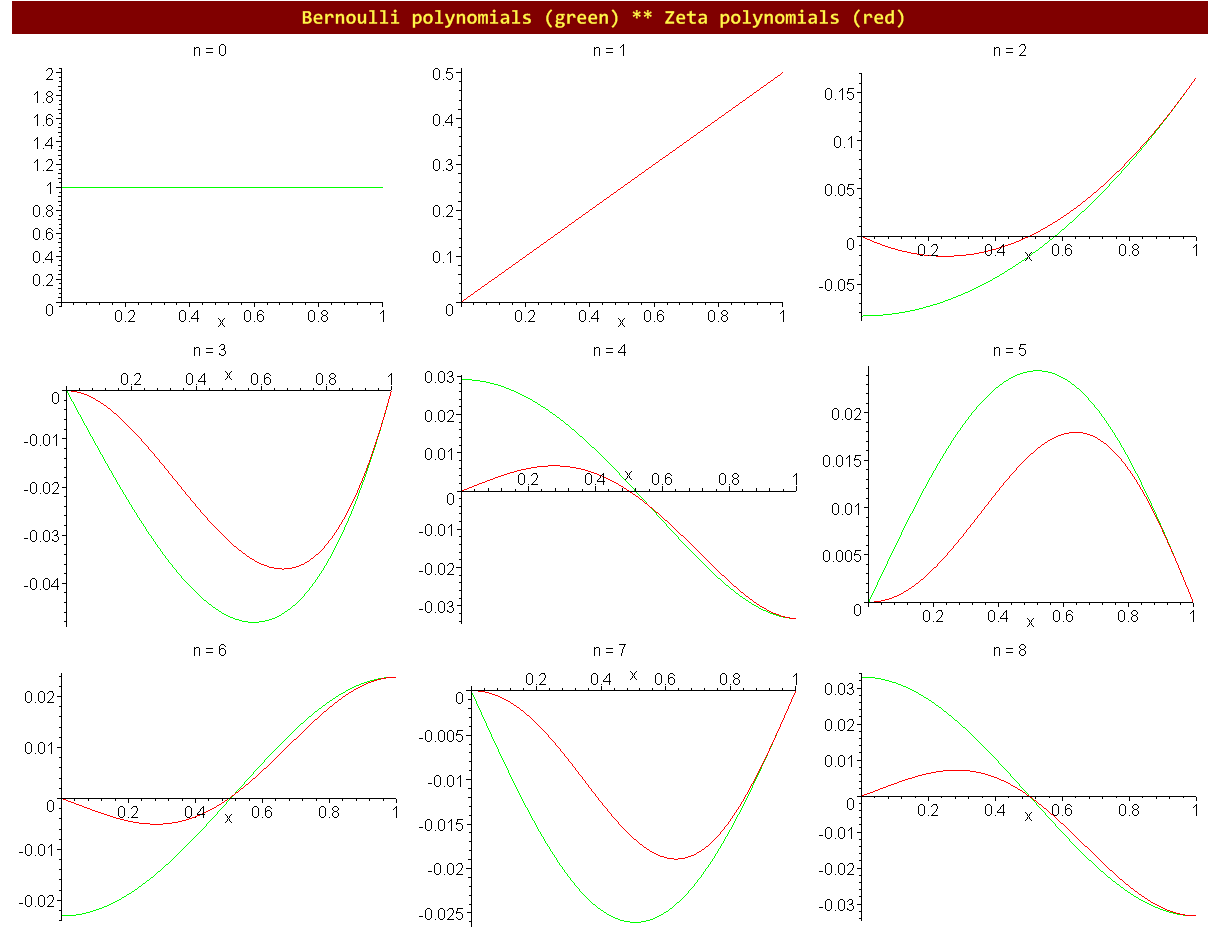### ::: Representations of the zeta polynomials :::

 ζ0(x) 1 / 1 ζ1(x) x / 2 ζ2(x) ( 2x2 − x ) / 6 ζ3(x) ( x3 − x2 ) / 4 ζ4(x) ( 6x4 − 9x3 + x2 + x ) / 30 ζ5(x) ( 2x5 − 4x4 + x3 + x2 ) / 12 ζ6(x) ( 6x6 − 15x5 + 6x4 + 6x3 − x2 − x ) / 42 ζ7(x) ( 3x7 − 9x6 + 5x5 + 5x4 − 2x3 − 2x2 ) / 24 ζ8(x) ( 10x8 − 35x7 + 25x6 + 25x5 − 17x4 − 17x3 + 3x2 + 3x ) / 90 ζ9(x) ( 2x9 − 8x8 + 7x7 + 7x6 − 7x5 − 7x4 + 3x3 + 3x2 ) / 20

We observe that for n ≥ 2 the polynomials xn / 2 − ζn(x) have factors, if n is even x(x+1) and if n is odd x2(x+1). This gives the following compact way to compute the zeta polynomials.

 ζn(x) = xn / 2 − (x2 + x) ρn−2(x) / cn   (n ≥ 2)
 ρn(x) cn+2 ρ0 1 6 ρ1 x 4 ρ2 9x2 − 1 30 ρ3 4x3 − x 12 ρ4 15x4 − 6x2 + 1 42 ρ5 9x5 − 5x3 + 2x 24 ρ6 35x6 − 25x4 + 17x2 − 3 90 ρ7 8x7 − 7x5 + 7x3 − 3x 20
# The first 10 zeta polynomials for the pocket calculator.
ZPR := proc(n, x)
if n=0 then RETURN(1)
elif n=1 then 0
elif n=2 then 1/3
elif n=3 then x/2
elif n=4 then (9*x^2-1)/15
elif n=5 then (4*x^2-1)*x/6
elif n=6 then (15*x^4-6*x^2+1)/21
elif n=7 then (9*x^4-5*x^2+2)*x/12
elif n=8 then (35*x^6-25*x^4+17*x^2-3)/45
elif n=9 then (8*x^6-7*x^4+7*x^2-3)*x/10
fi; (x^n - %*x*(x+1))/2 end;


### ::: Zeta polynomials versus Stirling polynomials :::

Close relatives of the Bernoulli polynomials are the Stirling polynomials0(x) is 1/x). For the definition of σn(x) we refer to GKP's Concrete Mathematics. Our definition differs from CM's by the factor n!.

for i from 0 to 7 do sigma[i] := unapply(sort(simplify(i!*expand(coeff(
convert(series((z*exp(z)/(exp(z)-1))^x,z,16),polynom),z,i)/x)),x),x) od;
σ0(x) = 1/x
σ1(x) = 1/2
σ2(x) = 1/4*x-1/12
σ3(x) = 1/8*x^2-1/8*x
σ4(x) = 1/16*x^3-1/8*x^2+1/48*x+1/120
σ5(x) = 1/32*x^4-5/48*x^3+5/96*x^2+1/48*x
σ6(x) = 1/64*x^5-5/64*x^4+5/64*x^3+13/576*x^2-1/96*x-1/252
σ7(x) = 1/128*x^6-7/128*x^5+35/384*x^4+7/1152*x^3-7/192*x^2-1/72*x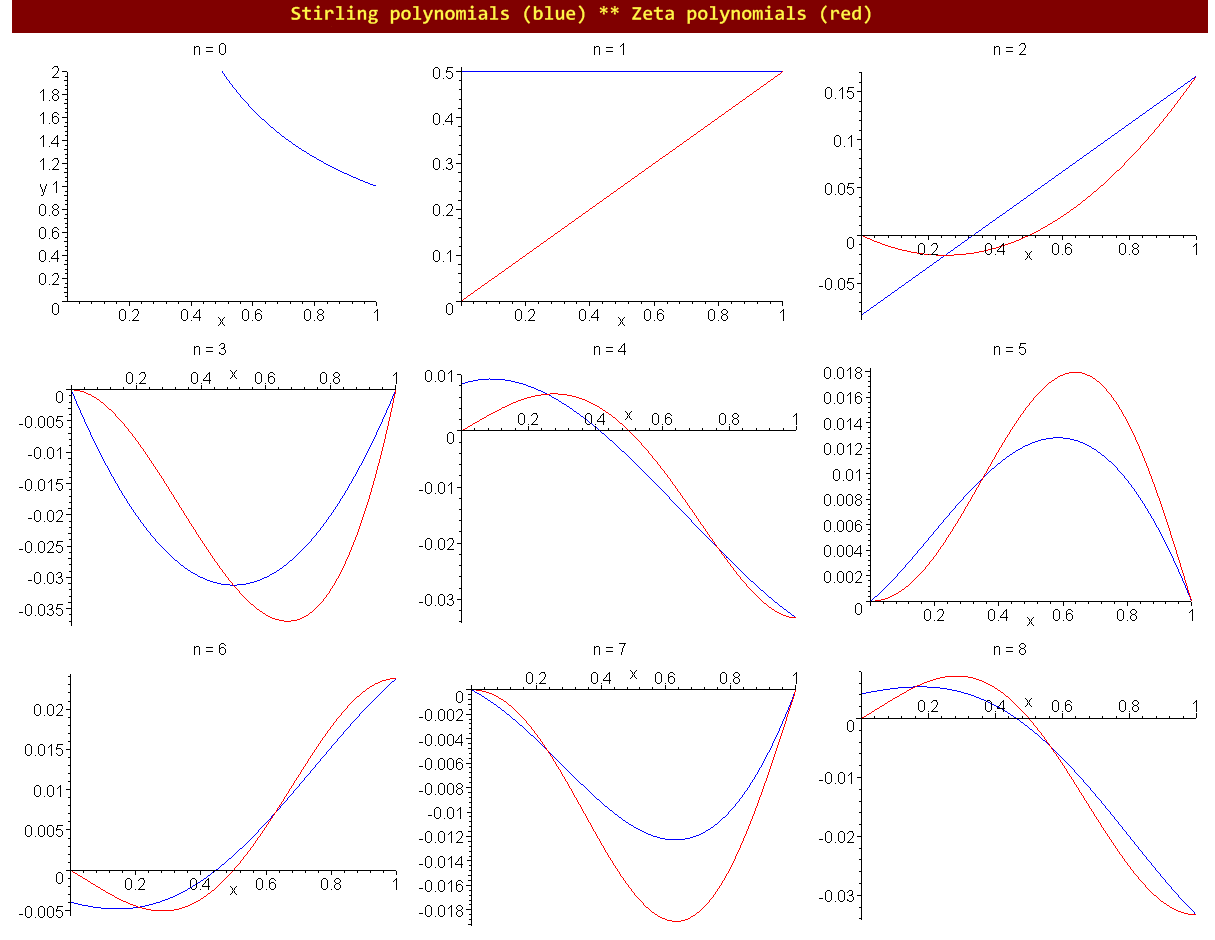### ::: The great polynomial shootout :::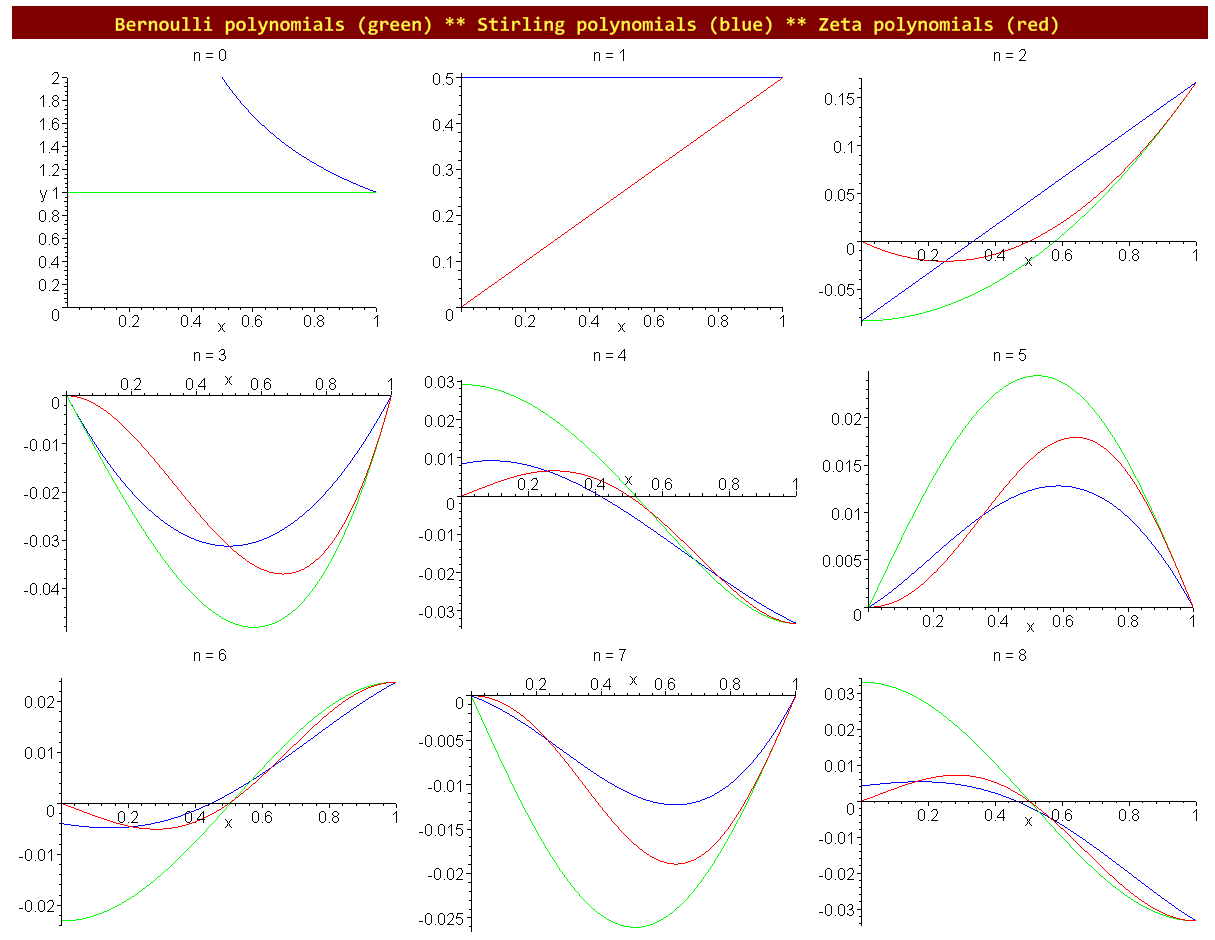Spieglein, Spieglein an der Wand, wer ist die Schönste im ganzen Land?

Our preliminary exploration of the zeta polynomials showed that they are members of a family of polynomial sequences which includes the Bernoulli and the Stirling polynomials. Some properties of the zeta polynomials indicate that they might be a useful addition to this family.

### ::: ... will the true zeta polynomials please stand up! :::

To bring this long story to an end, assume the Bernoulli numbers defined the right way, as Bn = Bn(1) (with B1 = 1/2). Then we could have also defined the zeta polynomials as(I)

I am quite fond of this formula, although a formula gourmet like Don Knuth might object that a sum should run from 0 to n − 1, not from 0 to n, so that we can apply the theory of definite summation. Therefore I count myself lucky that I could transform this identity by means of the delightful fact that ζn+1(1) = −(n+1)ζ(−n)  (n ≥ 0) into another cute formula(II)

On the right hand side ζ(z) denotes the Riemann zeta function. Clearly it is this representation which gave the polynomials their name. The proposition is that the definition I started from (zeta polynomials are transformed harmonic polynomials) agrees with this representation, that is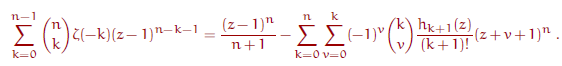(III)

This formula does have the form of a definite sum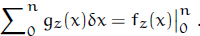Let us check (III) in the case n = 0. The left hand side is then an empty sum,  thus has by convention the value 0  for all z.  On the right hand side (z − 1)0 is 1, in the case z = 1 by the convention 00 = 1.  The double sum is 1, in the case z = −1 again by the convention 00 = 1.   Thus fz(0) = 0 for all z and the definite sum has the value fz(n).

Looking at z = 1 and remembering that hn(1) = n! H(n), H(n) the harmonic numbers, we find for n > 0 the special case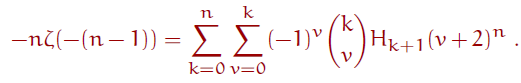(IV)

This is the formula representing the Bernoulli numbers as a harmonic sum which I wrote about in my last blog.

I searched in the literature but could not find the above formulas. Therefore I showed the zeta polynomials in the newsgroup de.sci.mathematik; however, no one could provide a reference. If you know a reference for these formulas, please let me know.

### ::: Recursive equation :::

A recursive equation for the zeta polynomials can now be easily derived. If we apply the standard recursion of the Bernoulli numbers to  formula (I) we get (using Iverson's bracket)(V)

### ::: New sequences for the OEIS :::

Based on the above considerations I consider to submit the following sequences to OEIS. Three of them refer directly or indirectly to the sequence A064538 which is in my opinion a core sequence.

» [P000000] The list of the coefficients of the zeta polynomials in descending order in the absolute form abs(ζn(x)) A064538(n).

           1
1, 0
2, 1, 0
1, 1, 0, 0
6, 9, 1, 1, 0
2, 4, 1, 1, 0, 0
6, 15, 6, 6, 1, 1, 0
3, 9, 5, 5, 2, 2, 0, 0


» [P000001] The list of the absolute coefficients of the ρn(x) polynomials, which are related to the zeta polynomials via the formula ζn(x) = (1/2) xn − (x2 + x) ρn−2(x) / A064538(n)  in descending order.

   1
   1   0
   9   0   1
   4   0   1   0
   15  0   6   0   1
   9   0   5   0   2   0
   35  0   25  0   17  0   3  0


» [P000002] The list of the coefficients of the  Riemann zeta polynomials RZn(x) = Sum_{k=0..n-1}(binom(n,k)Zeta(-k)pow(z-1,n-k-1) in descending order in the absolute form abs(RZn(x)) A064538(n).

              0
0, 1
0, 3, 2
0, 2, 3, 1
0, 15, 35, 25, 6
0, 6, 19, 21, 10, 2
0, 21, 84, 126, 91, 35, 6
0, 12, 58, 110, 107, 61, 21, 3


»  [P000003] Special values of of the zeta polynomials.

seq(abs(numer(ZP(2*i+1, -1/2))),i=0..11);
seq((1/3)numer(ZP(2*i+1,3/2)),  i=0..11);
seq((1/3)numer(euler(2*i+1,2)), i=0..11);

1, 3, 1, 11, 9, 233, 1819, 309867, 1067429, 73976863,...


» [P000004] Divisors of the denominator of the zeta polynomials.

seq(denom(ZP(i,x))/Clausen(i), i=0..20);
1, 1, 1, 2, 1, 6, 1, 12, 3, 10, 1, 12, 1,210,15,24,1,90,5,420,21,..


Implementation test.

ZetaPoly := proc(n,z) local k, power, Bernoulli;
Bernoulli := m -> bernoulli(m,1);
power := (u, v) -> if u=0 and v=0 then 1 else u^v fi:

for n from 0 to 8 do seq((n-1)*ZetaPoly(i,n),i=0..8) od;